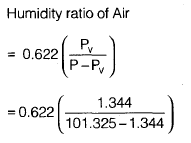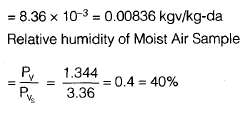Courses

# RAC And I.C. Engine - 2

## 20 Questions MCQ Test Mock Test Series - Mechanical Engineering (ME) for GATE 2020 | RAC And I.C. Engine - 2

Description
This mock test of RAC And I.C. Engine - 2 for GATE helps you for every GATE entrance exam. This contains 20 Multiple Choice Questions for GATE RAC And I.C. Engine - 2 (mcq) to study with solutions a complete question bank. The solved questions answers in this RAC And I.C. Engine - 2 quiz give you a good mix of easy questions and tough questions. GATE students definitely take this RAC And I.C. Engine - 2 exercise for a better result in the exam. You can find other RAC And I.C. Engine - 2 extra questions, long questions & short questions for GATE on EduRev as well by searching above.
*Answer can only contain numeric values
QUESTION: 1

### An industrial heat pump operates between the temperature of 27°C and –13°C. The rates of heat addition and heat rejection are 750 W and 1000 W, respectively. The COP for the heat pump is ____.

Solution: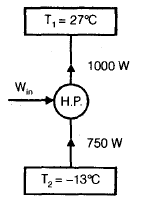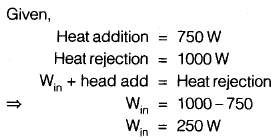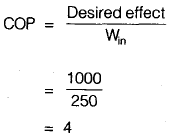QUESTION: 2

### In an ideal vapour compression refrigeration cycle, the specific enthalpy of refrigerant (in kJ/kg) at the following states is given as: Inlet of condenser : 283 Exit of condenser : 116 Exit of evaporator : 232 The COP of this cycle is

Solution: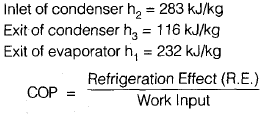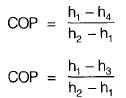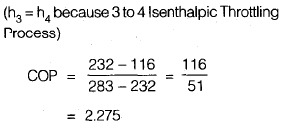QUESTION: 3

### In a cascade refrigeration system the COP of lower cycle is COP1 and that of upper cycle is COP2 , COP of combined cycle will be

Solution:
QUESTION: 4

A refrigerator operates between the temperature of –23°C and 27°C. If one TR = 3.5 kW, the minimum power required per TR to operate the refrigerator is

Solution: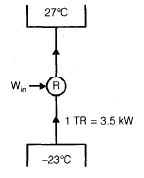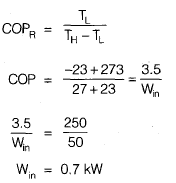*Answer can only contain numeric values
QUESTION: 5

In a vapour compression refrigeration plant, the refrigerant leaves the evaporator at 195 kJ/kg and the condenser at 65 kJ/kg. For 1 kg/s of refrigerant, the refrigeration effect is ___________ kW.

Solution: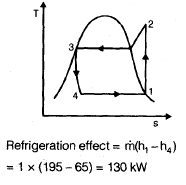QUESTION: 6

The wet bulb depression is zero, when relative humidity is equal to

Solution:
QUESTION: 7

The atmospheric air at 760 mm of Hg, dry bulb temperature 15°C and wet bulb temperature 11°C enters a heating coil whose temperature is 41°C. If the by-pass factor of the heating coil is 0.5, then what will be the dry bulb temperature of the air leaving the coil?

Solution: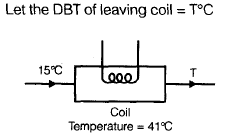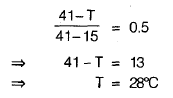QUESTION: 8

Water at 42°C is sprayed into a stream of air at atmospheric pressure, dry bulb temperature of 40°C and a wet bulb temperature of 20°C. The air leaving the spray humidifier is not saturated. Which of the following statements is true?

Solution:

Given, water spraying temperature = 42°C
Dry bulb temperature, Td = 40°C
Wet bulb temperature, Tw = 20°
Here we see that, water spraying temperature > Td

QUESTION: 9

During the adiabatic cooling of moist air

Solution: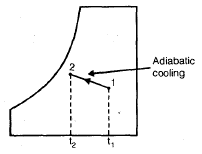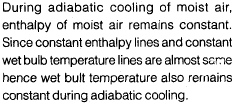QUESTION: 10

An air-conditioned room of volume 10 m3 has infiltration of air equivalent to 3 air changes per hour. Density of air is 1.2 kg/m3, specific heat Cp is 1 kJ/kgK and temperature difference between room and ambient air is 20 K. The sensible heat load due to infiltrated air is

Solution: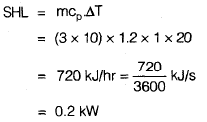QUESTION: 11

In diesel engine, the suction consists of

Solution:
QUESTION: 12

In petrol engine, ignition takes place

Solution:
QUESTION: 13

The ratio of shaft horse power to the energy supplied by the fuel is called

Solution:
QUESTION: 14

Indicated horse power of a four-stroke engine is given by

Solution:
QUESTION: 15

The expression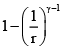denotes the following efficiencies of an I.C. engine

Solution:
QUESTION: 16

The compression ratio for a diesel engine as compared to petrol engine is

Solution:
QUESTION: 17

A moist air sample has dry bulb temperature of 30°C and specific humidity of 11.5 g water vapour per kg dry air. Assume molecular weight of air as 28.93. If the saturation vapour pressure of water at 30°C is 4.24 kPa and the total pressure is 90 kPa, then the relative humidity (in %) of air sample is

Solution: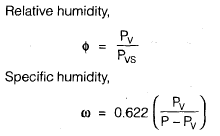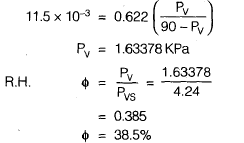QUESTION: 18

Atmospheric air at 1.013 bar and 35°C has relative humidity 60%. The saturation pressure of water vapour at 35°C is 5.628 kPa. The specific humidity of water vapour in kg vapour per kg dry air will be

Solution: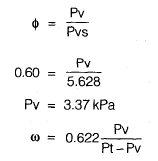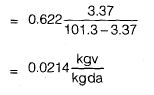QUESTION: 19

Moist air enters the cooling coil with mass flow rate of 10 kg-da/s at dry bulb temperature of 30°C and humidity ratio of 0.017 kg v/da. It leaves the cooling coil at dry bulb temperature of 16°C and humidity ratio of 0.008 kg v/da. If specific heat of humid air is 1.02 kJ/kg da K and latent heat of water vapour is 2500 kJ/kg v. The sensible and latent heat transfer of cooling coil are, respectively

Solution: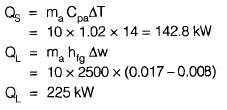QUESTION: 20

In a sample of moist air at saturated atmospheric pressure of 101.325 kPa and 26°C the partial pressure of water vapour is 1.344 kPa. If the saturation pressure of water vapour is 3.36 kPa at 26°C, then what are the humidity ratio and relative humidity of moist air sample?

Solution: